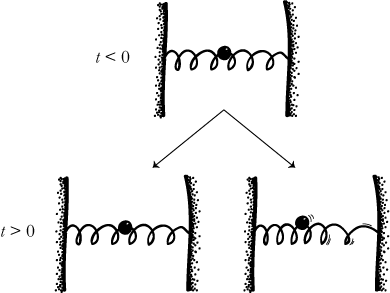# Another Unexpectedly Simple Failure of Determinism

Here’s a simple Newtonian system that fails to determine a unique Cauchy evolution, in the spirit of Norton’s dome. In the system I will describe, one begins with a mass sitting at rest. There are then two possible evolutions: the ball might just sit there for all of time, or it might start bouncing for no apparent reason.Take a spring with a mass in the middle that’s sitting at rest. Let’s set the center of the mass to be the center of our coordinate system x = 0. The differential equation describing the motion of this system (in a neighborhood of the origin) is that of a harmonic oscillator,

x” = -k2x,

where k is a constant describing the tension of the spring. Now, imagine that we wind up the spring very tightly, and then start letting it loose again, at a rate determined by the equation,

-k2 = 6/t2.

The equation of motion for this system is then given by,

x” = 6x/t2.

One obvious solution to this differential equation is x(t) = 0, at all times t. This represents an evolution in which the ball just sits there at rest, for all of time. However, it is easily shown that there is also a second solution:

x(t) = 0 for t ≤ 0
= t3 for t ≥ 0

So, determinism fails for this spring system: there is more than one Cauchy evolution for the initial conditions x = 0, t ≤ 0. It might be that the ball just sits there; or, it might be that the ball starts bouncing for times t > 0.What’s the pathology? (Left as a puzzle…)

Soul Physics is authored by Bryan W. Roberts. Thanks for subscribing.
Want more Soul Physics? Try the Soul Physics Tweet.

## One thought on “Another Unexpectedly Simple Failure of Determinism”

1.Bryan

This comment has been removed by the author.